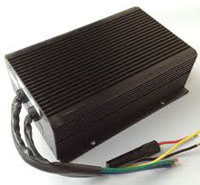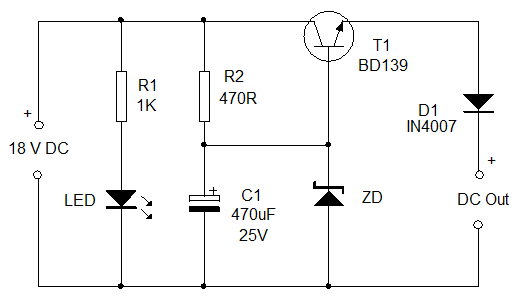# DC-DC Converter Types like Buck Converter and Boost Converter

A DC-DC converter is a device which accepts a DC input voltage and provides a DC output voltage. The output voltage can be greater than the input or vice versa. These are used to match the loads to the power supply. The simplest DC-DC converter circuit consists of a switch which controls the connection and disconnection of load to the power supply.A basic DC-DC converter consists of energy transferred from load to the energy storage devices like inductors or capacitor through switches like a transistor or a diode. They can be used as linear voltage regulators or switched mode regulators. In a linear voltage regulator, the base voltage of a transistor is driven by a control circuit to obtain the desired output voltages. In a switched mode regulator, transistor is used as a switch. In a step down converter or a buck converter, when switch is closed, the inductor allows current to flow to the load and when the switch is opened, the inductor supplies the stored energy to the load.

3 Categories of DC to DC Converter

• Buck converters
• Boost converters
• Buck boost converters

Buck Converters: The buck converters are used to convert the high input voltage to low output voltage. In this converter continuous output current gives the less output voltage ripples.

Boost Converters: The boost converters are used to convert the lower input voltage to higher output voltage. In a step up converter or a boost converter, when switch is closed, the load gets voltage supply from the capacitor which charges through the current passing through the inductor and when switch is open, the load gets supply from the input stage and the inductor.

Buck Boost Converters: In buck boost converter, the output can be maintained higher or lower, which depends on the source voltage. When the source voltage is high then output voltage is low and source voltage is low then output voltage is high.

### Boost Converters

Here brief details of the boost converter are discussed below

The Boost Converter is a simple converter. It is used to convert a DC voltage from lower level to higher level. Boost Converter is also called a DC to DC converter. The Boost Converters (DC-DC Converters) were developed in the early 1960s. These converters are designed using semiconductors switching devices.

• Without using the Boost Converter: In semiconductor switching devices, the Linear regulated circuits (DC power regulated circuits) access voltage from the unregulated input supply (AC power supply) and due to this there is a power loss. The power loss is proportional to the voltage drop.
• Using the Boost Converters: In switching devices, the converters converts the unregulated AC or DC input voltage to regulated DC output voltage.

Most of the Boost converters are used in SMPS devices. The SMPS with input supply access from the AC mains, the input voltage is rectified and filtered using a capacitor and rectifier.

### Working Principle of Boost Converters:

Electrical power circuit designers mostly choose the boost mode converter because the output voltage is always high when compared to source voltage.

1. In this circuit power stage can be operates in two modes Continuous Conduction Mode (CCM).
2.  Discontinuous Conduction Mode (DCM).

#### 1. Continuous Conduction Mode:

The Boost Converter Continuous Switching Mode is constructed with given components that are inductor, capacitor and input voltage source and one switching device. In this inductor acts as a power storage element. The boost converter switch is controlled by the PWM (pulse width modulator). When switch is ON the energy is developed in the inductor and more energy is delivered to the output. It is possible to convert high voltage capacitors from low voltage input source. The input voltage is always greater than the output voltage. In continuous conduction mode, the current is increased with respect to input voltage.

#### 2. Discontinuous Conduction Mode:

The discontinuous conduction mode circuit is build with inductor, capacitor, switching device and input voltage sourceInductor is a power storage element same as continuous conduction mode. In discontinuous mode, when the switch is ON the energy is delivered to the inductor. And if the switch is OFF some period of time the inductor current reaches to zero when next switching cycle is on. The output capacitor is charging and discharging with respect to input voltage. The output voltage is less than compared to the continuous mode.

• Gives the high output voltage
• Low operating duty cycles
• Lower voltage on MOSFET
• Output voltage with low distortion
• Good quality of wave forms even the line frequency is present

Applications:

• Automotive applications
• Power amplifier applications
• Battery power systems
• Consumer Electronics
• Communication Applications Battery Charging circuits
• In heaters and welders
• DC motor drives
• Power factor correction circuits
• Distributed power architecture systems

### Working Example of a DC-DC converters

Presenting here a simple DC-DC converter circuit to power various DC operated circuits. It can provide DC power supply up to 18 volts DC. You can simply select the output voltage by changing the value of the Zener diode ZD. The circuit has both voltage and current regulation.

### Circuit Components:

• An LED
• A 18V battery
• Zener diode which is used as a voltage regulator
• A transistor which works as a switch.

System Working:Input voltage for the circuit is obtained from an 18 volt 500 mA transformer based power supply. You can also use input voltage from a battery. The 18 volts DC from the power supply is given to the collector and base of the Medium power transistor BD139 (T1). Resistor R1 limits the base current of T1 so that the output voltage will be current regulated.

Zener diode ZD regulates the output voltage. Select the appropriate value of Zener to fix the output voltage. For example, if the Zener diode is a 12 volt one, the circuit gives 12 volts DC at the output. Diode D1 is used as a polarity protector.LED provides power on status. Here we have used a DC-DC converter in linear mode where the base voltage to the transistor is controlled to get the desired output, depending on the Zener diode voltage.

I hope you have clearly understand the topic of the types of DC-DC converter and there types. If you have any queries on this topic or on the electrical and electronic projects leave the comments below.